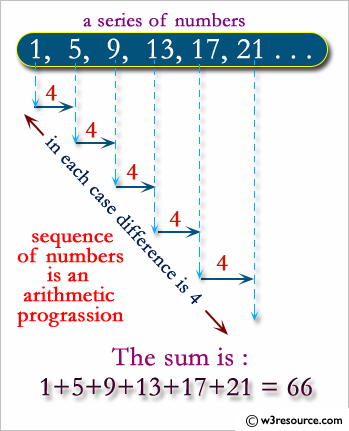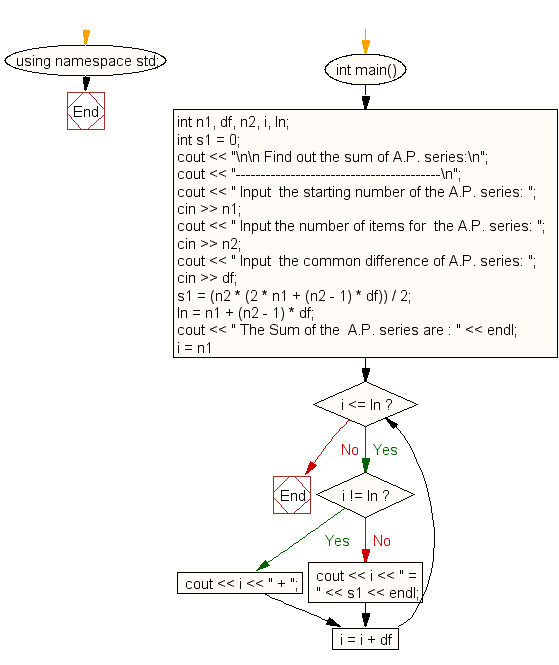﻿ C++ Exercises: Find out the sum of an A.P. series - w3resource# C++ Exercises: Find out the sum of an A.P. series

## C++ For Loop: Exercise-31 with Solution

Write a program in C++ to find out the sum of an A.P. series.

Pictorial Presentation:Sample Solution:-

C++ Code :

``````#include <iostream>
using namespace std;

int main()
{
int n1, df, n2, i, ln;
int s1 = 0;
cout << "\n\n Find out the sum of A.P. series:\n";
cout << "-----------------------------------------\n";
cout << " Input  the starting number of the A.P. series: ";
cin >> n1;
cout << " Input the number of items for  the A.P. series: ";
cin >> n2;
cout << " Input  the common difference of A.P. series: ";
cin >> df;
s1 = (n2 * (2 * n1 + (n2 - 1) * df)) / 2;
ln = n1 + (n2 - 1) * df;
cout << " The Sum of the  A.P. series are : " << endl;
for (i = n1; i <= ln; i = i + df)
{
if (i != ln)
cout << i << " + ";
else
cout << i << " = " << s1 << endl;
}
}
``````

Sample Output:

``` Find out the sum of A.P. series:
-----------------------------------------
Input  the starting number of the A.P. series: 1
Input the number of items for  the A.P. series: 8
Input  the common difference of A.P. series: 5
The Sum of the  A.P. series are :
1 + 6 + 11 + 16 + 21 + 26 + 31 + 36 = 148
```

Flowchart:C++ Code Editor:

Contribute your code and comments through Disqus.

What is the difficulty level of this exercise?

﻿